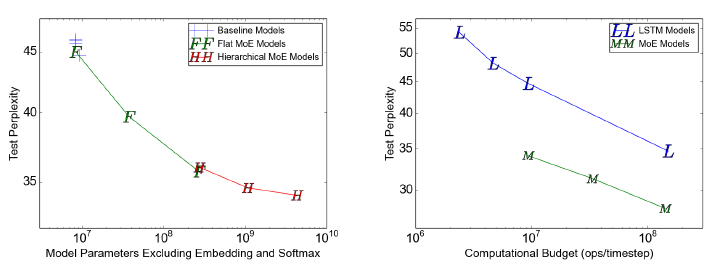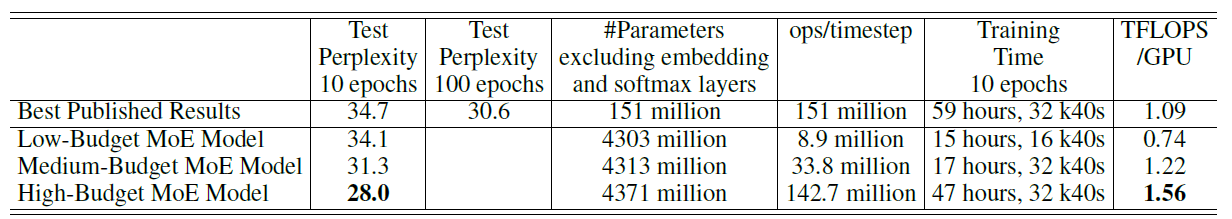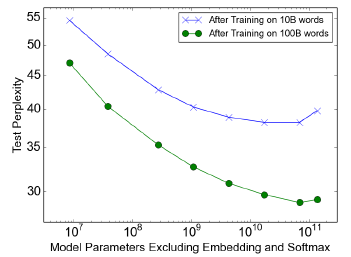# Review: Outrageously Large Neural Networks: The Sparsely-Gated Mixture-of-Experts Layer (MoE)

## Using MoE, Outperforms GNMT and Deep-Att

In this story, Outrageously Large Neural Networks: The Sparsely-Gated Mixture-of-Experts Layer, (MoE), by Google Brain, and Jagiellonian University, is briefly reviewed. This is a paper by Prof. Hinton’s Group. In this paper:

• Sparsely-Gated Mixture-of-Experts layer (MoE) is designed, consisting of up to thousands of feed-forward sub-networks, achieving greater than 1000× improvements in model capacity with only minor losses in computational efficiency on modern GPU clusters.

This is a paper in 2017 ICLR with over 700 citations. (Sik-Ho Tsang @ Medium)

# Outline

1. Sparsely-Gated Mixture-of-Experts Layer (MoE)
2. Experimental Results

# 1. Sparsely-Gated Mixture-of-Experts Layer (MoE)

## 1.1. MoE Layer

• The Mixture-of-Experts (MoE) layer consists of a set of n “expert networks” E1, …, En, and a “gating network” G whose output is a sparse n-imensional vector.
• Each expert has separate parameters.
• Let us denote by G(x) and Ei(x) the output of the gating network and the output of the i-th expert network for a given input x. The output y of the MoE module is:
• Wherever G(x)i = 0, we need not compute Ei(x) to save the computation.

## 1.2. Hierarchical MoE Layer

• If the number of experts is very large, we can reduce the branching factor by using a two-level hierarchical MoE. In a hierarchical MoE, a primary gating network chooses a sparse weighted combination of “experts”, each of which is itself a secondary mixture-of-experts with its own gating network.
• The primary gating network is Gprimary, the secondary gating networks are (G1, G2, …, Ga), and the expert networks are (E0,0, E0,1, …, Ea,b). The output of the MoE is given by:

## 1.2. MoE Expert

• A MoE whose experts have one hidden layer is similar to the block-wise Dropout, where the dropped-out layer is sandwiched between fully-activated layers.

## 1.3. Gating

• The gating is to multiply the input by a trainable weight matrix Wg and then apply the Softmax function.
• Before taking the softmax function, tunable Gaussian noise is added, then only the top k values are kept. Others are set to -∞.
• where the amount of noise per component is controlled by a second trainable weight matrix Wnoise.

## 1.4. Mixing Data Parallelism and Model Parallelism

The goal to train a trillion-parameter model on a trillion-word corpus.

• If the gating network chooses k out of n experts for each example, then for a batch of b examples, each expert receives a much smaller batch of approximately kbn << b examples.
• If the model is distributed over d devices, and each device processes a batch of size b, each expert receives a batch of approximately kbd/n examples. Thus, a factor of d improvement in expert batch size is achieved.
• This technique allows to increase the number of experts (and hence the number of parameters) by proportionally increasing the number of devices in the training cluster.
• The total batch size increases, keeping the batch size per expert constant.

## 2.1. 1 Billion Word Language Modeling

• MoE Models: The proposed models consist of two stacked LSTM layers with a MoE layer between them.
• Models are trained with flat MoEs containing 4, 32, and 256 experts, and with hierarchical MoEs containing 256, 1024, and 4096 experts.
• Each expert had about 1 million parameters.
• For all the MoE layers, 4 experts were active per input.Model comparison on 1-Billion-Word Language-Modeling Benchmark
• Left: The model with 4 always-active experts performed (unsurprisingly) similarly to the computationally-matched baseline models, while the largest of the models (4096 experts) achieved an impressive 24% lower perplexity on the test set.
• Right: Compared with LSTM models, MoE models achieve lower perplexity with similar computational budget.Summary of high-capacity MoE-augmented models with varying computational budgets, vs. best previously published results
• For the baseline models with no MoE, observed computational efficiency ranged from 1.07–1.29 TFLOPS/GPU.
• For the proposed low-computation MoE models, computation efficiency ranged from 0.74-0.90 TFLOPS/GPU, except for the 4-expert model which did not make full use of the available parallelism.
• The highest-computation MoE model was more efficient at 1.56 TFLOPS/GPU, likely due to the larger matrices.

## 2.2. 100 Billion Word Google News CorpusLanguage modeling on a 100 billion word corpus
• When training over the full 100 billion words, test perplexity improves significantly up to 65536 experts (68 billion parameters), dropping 39% lower than the computationally matched baseline, but degrades at 131072 experts, possibly a result of too much sparsity.

## 2.3. Machine Translation

• MoE model used here was a modified version of the GNMT.
• To reduce computation, the number of LSTM layers in the encoder and decoder are decreased from 9 and 8 to 3 and 2 respectively.
• MoE layers are inserted in both the encoder (between layers 2 and 3) and the decoder (between layers 1 and 2). Each MoE layer contained up to 2048 experts each with about two million parameters, adding a total of about 8 billion parameters to the models.

The proposed approach achieved BLEU scores of 40.56 and 26.03 on the WMT’14 En>Fr and En>De benchmarks, outperforms GNMT and Deep-Att.

On the Google Production dataset, MoE model achieved 1.01 higher test BLEU score even after training for only one sixth of the time.

The MoE model achieves 19% lower perplexity on the dev set than the multilingual GNMT model.

• On BLEU score, the MoE model significantly beats the multilingual GNMT model on 11 of the 12 language pairs (by as much as 5.84 points), and even beats the monolingual GNMT models on 8 of 12 language pairs.

## Natural Language Processing (NLP)

Language/Sequence Model: 2007 [Bengio TNN’07] 2013 [Word2Vec] [NCE] [Negative Sampling] 2014 [GloVe] [GRU] [Doc2Vec] 2015 [Skip-Thought] 2016 [GCNN/GLU] [context2vec] [Jozefowicz arXiv’16] [LSTM-Char-CNN] 2017 [TagLM] [CoVe] [MoE]
Machine Translation: 2014 [Seq2Seq] [RNN Encoder-Decoder] 2015 [Attention Decoder/RNNSearch] 2016 [GNMT] [ByteNet] [Deep-ED & Deep-Att] 2017 [ConvS2S] [Transformer] [MoE]
Image Captioning: 2015 [m-RNN] [R-CNN+BRNN] [Show and Tell/NIC] [Show, Attend and Tell]

--

--